﻿ Column Algorithm

# Column Algorithm

A Column Algorithm is a data block calculated with a formula based on other data blocks of the Layout.

The following example is a report with a Layout having three data blocks, block (a) is Sales Amount, block (b) is Sales Amount of the previous year, and block (c) is the percentage of variation, a column algorithm calculated with the formula  (a-b)/b*100.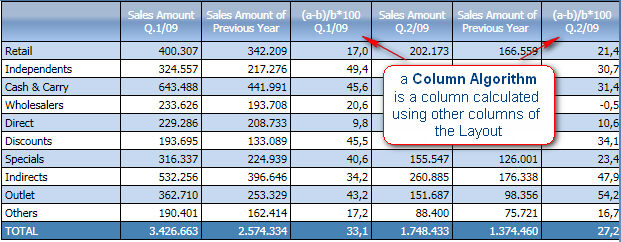The formula uses the block letters to refer to other blocks and can refer to other Column Algorithms. See below for a list of operators.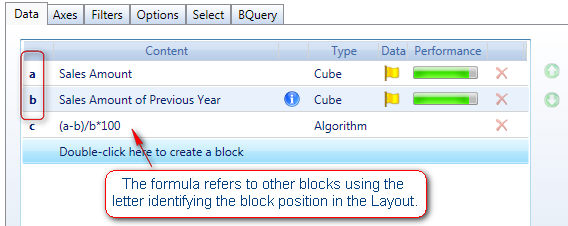A formula may return different data types : Numeric, Text, Date and Picture. The default type is numeric, otherwise select the appropriate option from the data type drop-down as shown.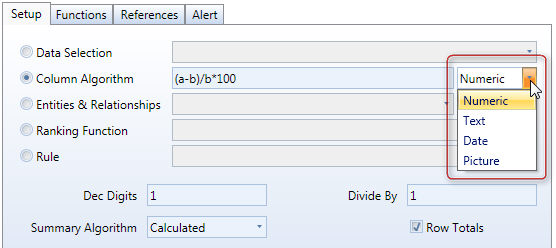The Picture option, (applicable to the objects DataView, Label and Button), allows to display a images in the report cells. The formula should return the name of a picture file and by selecting the Picture data type option the image is displayed. The images files must be embedded into the Capsule, to import an image, go to Capsule icon of the ribbon bar, select Capsule Properties and then Capsule Images. The supported file formats are JPG, GIF, BMP, PNG (it is recommended to use compressed formats such as JPG or GIF to avoid creating large Capsule files).

Example of a DataView with column algorithms displaying images.

The following table lists the operators which can be used in formulas

 Type Operator Description Example Arithmetic + Addition a+b - Subtraction a-b / Division a/b * Multiplication a*b % Percentage a/b% is equivalent to a/b*100 a*(1+15%) ^ Exponentiation a^2 is equal to a*a Comparison = Equal to a=b > Greater than a>b < Less than a= Greater than or equal to a>=b <= Less than or equal to a<=b <> Not equal to a<>b Text & Concatenation a&b concatenates the two text strings. a&”myText” concatenates myText with the string contained in column a. When using this operator, the result of the algorithm is a text string therefore you must select the option Text from the data type list box. left(text,num_char) Left substring Returns the leftmost characters from a text string.   Left(a,4) returns the first four characters of the text contained in block (a) right(text,num_char) Right substring Returns the rightmost characters from a text string. Right(a,4) returns the last four characters of the text contained in block (a) mid(text,start_pos,num_char) Substring Returns the specified number of characters from a text string starting from the specified position..   Mid(a,2,4) returns the four characters starting from position 2 of the text contained in block (a)

Here the list of functions which can be used in formulas.

ABS(number ). Returns the absolute value of a number.

ABS(a-b)

AND(logical conditions list). Returns True if all conditions are True; returns False if at least one condition evaluates to false.

AND(a>0,b<100)

returns true if the value in column a is greater than zero and the value in column b is lesser than 100.

OR( logical conditions list). Returns True if at least one condition is True; returns False if all conditions evaluate to False.

OR(a>0,b<100)

returns true if the value in column a is greater than zero or if the value in column b is lesser than 100.

IF (condition, true_value, false_value ). condition any logical expression, true_value is the value to return if the condition is true and false_value if false.

if((a*b)>0,a,c)

if(a>100,”Greater”, ”r;Less”)

if(and(a>0,b>0),a*b,0)

Today( ). Returns the current date.

today()-a

if a is a date InfoCube, returns the number of days between the date in column a and the current date. For example, if a is a date InfoCube storing delivery dates, you can calculate the number of days between the current date and the delivery date.

today()+a

if a is a numeric InfoCube, returns the date corresponding to the current date plus the number of days specified in column a. For example, if a is and InfoCube storing the delivery lead-time, you can calculate the date of delivery adding the lead-time to the current date.

dt(column). Returns the column total. To use this function, the Layout must have an entity set by row.

a/dt(a)*100

rt(column). Returns the row total. To use this function, the Layout must have an entity set by column.

a/rt(a)*100

gt(column). Returns the grand-total. To use this function, the Layout must have an entity set by row and by column.

a/gt(a)*100

Note:

The above examples use the comma as list separator. The list separator character may vary depending on your computer’s settings. The most common characters used are the comma and the semicolon. The list separator is defined in your computer’s Control Panel / Regional settings window.

## What's an Expression

A Board Expression is a saved column algorithm block.

To create an Expression, go in edit mode, open a layout and create a column algorithm block,give ti a Heading, then click on the "Save as Expression" icon as shown in figure.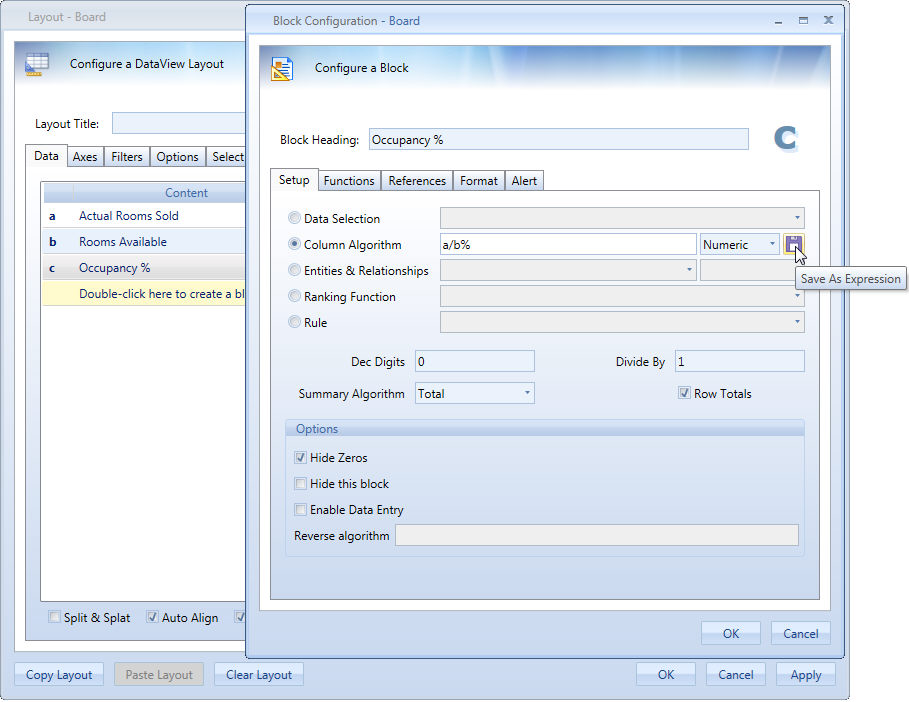Figure 1

The expression will be saved in the cubes list into the Expressions Group, name of the Expression Cube will be the same as Block Heading as shown in figure.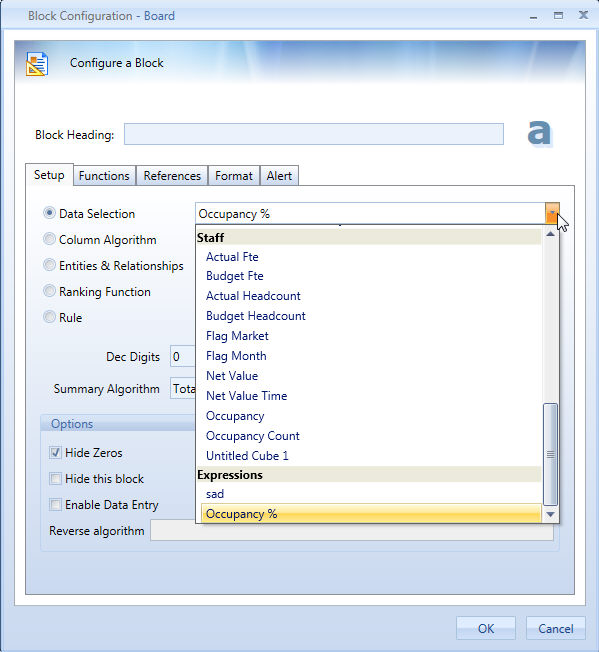Figure 2

To edit an expression, just create another one and save it with the same name, it's strictly recommended to keep a capsule containing all the layout used to create expression. To delete an expression just go in database manager-> cubes, you will see all the expressions in the cube list, click on delete to remove the selected one.

## Usage

Once the expression gets created, it will be available to be used in every layout. It's not necessary to have blocks used for Expression calculation in the same layout in order to use it, the expression can even be the only block present in the layout. It will be shown as a data block.

Expressions will work on the same entities of the original cubes used to create them, expressions totals are always calculated, this means that leaf elements do not sum to obtain totals.

Expressions can't be used in the Procedure Command "If then ... else" and "Select based on ..."

#### Example

Let's suppose that a hospitality company wants to know the occupancy percentage of its Hotels for each city. The company has two cubes:

a. Rooms Available

b. Rooms sold

The Occupancy percentage cube will be an expression as shown in Figure 1.

The obtained report will look like the following one: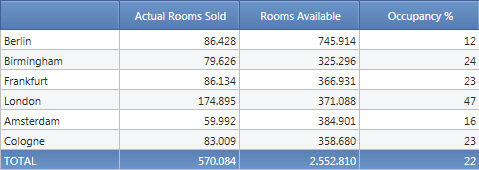Now let's suppose they want to know the Occupancy % by region, it will be sufficient to create a layout with a single Expression Block Occupancy % (Figure 2) and put regions in row: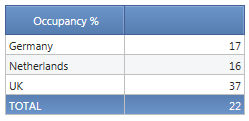The two advantages of this approach are:

• It's not needed anymore to create 3 blocks to have this information
• Occupancy ratio of higher level (Region) is not the sum of the leaf level (City) but gets calculated every time taking data from the two cubes used to create the Expression.

Note:

Expressions do not support functions, referto and totalby. Only exception is previous year that will be applied to all the cubes in the formula.